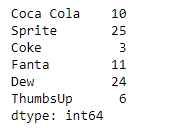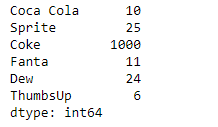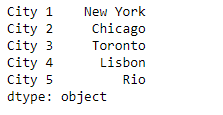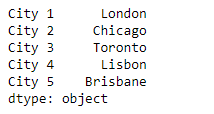# Python | Pandas Series.replace()

• Last Updated : 10 Feb, 2019

Pandas series is a One-dimensional ndarray with axis labels. The labels need not be unique but must be a hashable type. The object supports both integer- and label-based indexing and provides a host of methods for performing operations involving the index.

Pandas` Series.replace()` function is used to replace values given in to_replace with value. The values of the Series are replaced with other values dynamically.

Syntax: Series.replace(to_replace=None, value=None, inplace=False, limit=None, regex=False, method=’pad’)

Parameter :
to_replace : How to find the values that will be replaced.
value : Value to replace any values matching to_replace with.
inplace : If True, in place.
limit : Maximum size gap to forward or backward fill.
regex : Whether to interpret to_replace and/or value as regular expressions
method : The method to use when for replacement, when to_replace is a scalar, list or tuple and value is None.

Returns : Object after replacement.

Example #1: Use `Series.replace()` function to replace some values from the given Series object.

 `# importing pandas as pd``import` `pandas as pd`` ` `# Creating the Series``sr ``=` `pd.Series([``10``, ``25``, ``3``, ``11``, ``24``, ``6``])`` ` `# Create the Index``index_ ``=` `[``'Coca Cola'``, ``'Sprite'``, ``'Coke'``, ``'Fanta'``, ``'Dew'``, ``'ThumbsUp'``]`` ` `# set the index``sr.index ``=` `index_`` ` `# Print the series``print``(sr)`

Output :Now we will use `Series.replace()` function to replace the old values with the new ones.

 `# replace 3 by 1000``result ``=` `sr.replace(to_replace ``=` `3``, value ``=` `1000``)`` ` `# Print the result``print``(result)`

Output :As we can see in the output, the `Series.replace()` function has successfully replaced the old value with the new one.

Example #2 : Use `Series.replace()` function to replace some values from the given Series object.

 `# importing pandas as pd``import` `pandas as pd`` ` `# Creating the Series``sr ``=` `pd.Series([``'New York'``, ``'Chicago'``, ``'Toronto'``, ``'Lisbon'``, ``'Rio'``])`` ` `# Create the Index``index_ ``=` `[``'City 1'``, ``'City 2'``, ``'City 3'``, ``'City 4'``, ``'City 5'``] `` ` `# set the index``sr.index ``=` `index_`` ` `# Print the series``print``(sr)`

Output :Now we will use `Series.replace()` function to replace the old values with the new ones using a list.

 `# replace the old ones in the list with ``# the new values``result ``=` `sr.replace(to_replace ``=` `[``'New York'``, ``'Rio'``], value ``=` `[``'London'``, ``'Brisbane'``])`` ` `# Print the result``print``(result)`

Output :As we can see in the output, the `Series.replace()` function has successfully replaced the old value with the new one using the list.

My Personal Notes arrow_drop_up